Related Articles

# Replace NumPy array elements that doesn’t satisfy the given condition

• Last Updated : 25 Oct, 2020

Sometimes in Numpy array, we want to apply certain conditions to filter out some values and then either replace or remove them. The conditions can be like if certain values are greater than or less than a particular constant, then replace all those values by some other number.

For this, we can use Relational operators like ‘>’, ‘<‘, etc and other functions like numpy.where().

Method 1: Using Relational operators

Example 1: In 1-D Numpy array

## Python3

 `# Importing Numpy module``import` `numpy as np`` ` `# Creating a 1-D Numpy array``n_arr ``=` `np.array([``75.42436315``, ``42.48558583``, ``60.32924763``])``print``(``"Given array:"``)``print``(n_arr)`` ` `print``(``"\nReplace all elements of array which are greater than 50. to 15.50"``)``n_arr[n_arr > ``50.``] ``=` `15.50`` ` `print``(``"New array :\n"``)``print``(n_arr)`

Output: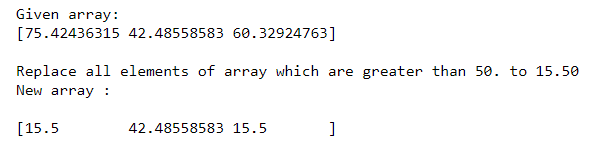In the above question, we replace all values greater than 50 with 15.50 in 1-D Numpy array.

Example 2(A): In 2-D Numpy array

## Python3

 `# Importing Numpy module``import` `numpy as np`` ` `# Creating a 2-D Numpy array``n_arr ``=` `np.array([[``45.42436315``, ``52.48558583``, ``10.32924763``],``                  ``[``5.7439979``, ``50.58220701``, ``25.38213418``]])``print``(``"Given array:"``)``print``(n_arr)`` ` `print``(``"\nReplace all elements of array which are greater than 30. to 5.25"``)``n_arr[n_arr > ``30.``] ``=` `5.25`` ` `print``(``"New array :\n"``)``print``(n_arr)`

Output: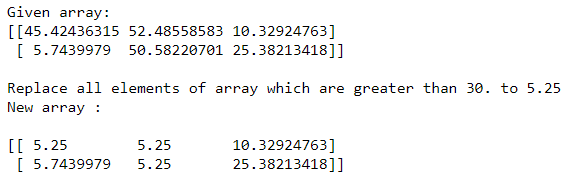In the above question, we replace all values greater than 30 with 5.25 in 2-D Numpy array.

Example 3: In 3-D Numpy array

## Python3

 `# Importing Numpy module``import` `numpy as np`` ` `# Creating a 3-D Numpy array``n_arr ``=` `np.array([[[``11``, ``25.5``, ``70.6``], [``30.9``, ``45.5``, ``55.9``], [``20.7``, ``45.8``, ``7.1``]],``                  ``[[``50.1``, ``65.9``, ``8.2``], [``70.4``, ``85.8``, ``10.3``], [``11.3``, ``22.2``, ``33.6``]],``                  ``[[``19.9``, ``69.7``, ``36.8``], [``1.2``, ``5.1``, ``24.4``], [``4.9``, ``20.8``, ``96.7``]]])`` ` `print``(``"Given array:"``)``print``(n_arr)`` ` `print``(``"\nReplace all elements of array which are less than 10 to Nan"``)``n_arr[n_arr < ``10.``] ``=` `np.nan`` ` `print``(``"New array :\n"``)``print``(n_arr)`

Output: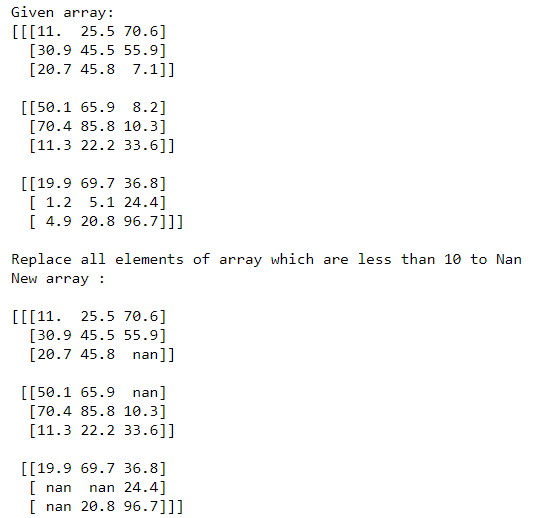In the above question, we replace all values less than 10 with Nan in 3-D Numpy array.

Method 2: Using numpy.where()

It returns the indices of elements in an input array where the given condition is satisfied.

Example 1:

## Python3

 `# Importing Numpy module``import` `numpy as np`` ` `# Creating a 2-D Numpy array``n_arr ``=` `np.array([[``45``, ``52``, ``10``],``                  ``[``1``, ``5``, ``25``]])`` ` `print``(``"Given array:"``)``print``(n_arr)`` ` `print``("\nReplace ``all` `elements of array which are \``greater than ``or` `equal to ``25` `to ``0``")`` ` `print``(``"else remains the same "``)``print``(np.where(n_arr >``=` `25``, ``0``, n_arr))`

Output: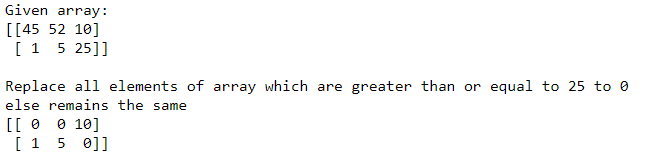In the above question, we replace all values greater than or equal to 25 with 0, else remain the same.

Example 2:

## Python3

 `# Importing Numpy module``import` `numpy as np`` ` `# Creating a 2-D Numpy array``n_arr ``=` `np.array([[``45``, ``52``, ``10``],``                  ``[``1``, ``5``, ``25``],``                  ``[``50``, ``40``, ``81``]])`` ` `print``(``"Given array:"``)``print``(n_arr)`` ` `print``("\nReplace ``all` `elements of array which are \``less than ``or` `equal to ``25` `with Nan")`` ` `print``(``"else with 1 "``)``print``(np.where(n_arr <``=` `25``, np.nan, ``1``))`

Output: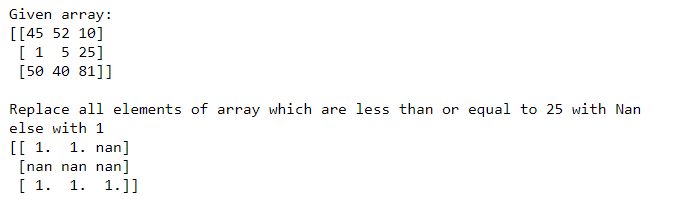In the above question, we replace all values less than or equal to 25 with Nan, else with 1.

Attention geek! Strengthen your foundations with the Python Programming Foundation Course and learn the basics.

To begin with, your interview preparations Enhance your Data Structures concepts with the Python DS Course. And to begin with your Machine Learning Journey, join the Machine Learning – Basic Level Course

My Personal Notes arrow_drop_up